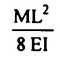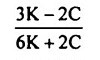Search

# Strength of Materials(181-200)

Updated: Apr 28, 2020

181. Consider the following statements:

If two planes at right angles carry only shear stress of magnitude 'q', then the

1. centre of the Mohr's circle would lie at the origin

2. angle between the principal plane and the plane of maximum shear would be

equal to 45°

182. A bar of square section is subjected to a pull of 10,000 kg. If the maximum

allowable shear stress on any section is 500 kg/cm², then the side of the square

section will be √15

183. According to Tresca, yield locus is a/ an circle

184. The principal stresses at a point in an elastic material are 1.5 σ (tensile), a

(tensile), and σ/2 (compressive). If the elastic limit in simple tension is 2000

kg/cm² and the Poisson's ratio is 0.30, then the value of a at failure would work

out to be 1000 kg/cm² according to maximum strain theory

185. A beam simply supported at both the ends, of length 'L' carries two equal unlike couples M at two ends. If the flexural rigidity, El ••= constant, then the central deflection of beam is given by186. If the crushing stress in the material of a mild steel column is 3300 kg/cm², Euler's formula for crippling load is applicable for slenderness ratio equal to /greater than 80

187. Which one of the following pairs is not correctly matched ?188. The resultant cuts the base of a circular column of diameter 4d' with an

eccentricity equal to d/4. The ratio between the maximum compressive stress

and the maximum tensile stress is 5

189.A three hinged parabolic arch rib of span L' and crown rise 4h' carries a uniformly distributed superimposed load of intensity 'w' per unit length. The hinges are located on two abutments at the same level and the third hinge at a quarter span location from left hand abutment. The horizontal

thrust on the abutment is wL²/12h

190. A shaft is subjected to a bending moment M and a torque T. The equivalent bending moment 'Mₑᵩ' on the shaft is given by191. Consider the following statements for a rectangular footing of length '/' and1. The stresses developed at points A and D are equal.

2. The stress developed at point B is maximum.

3. The stresses developed at point B and C are compressive.

192. A 2m long bar of uniform section 50 mm² extends 2 mm under a limiting axial stress of 200 N/mm². What is the modulus of resilience for the bar ? 0.10

If E=2.06 x 105 N/mm², an axial pull of 60 kN suddenly applied to a steel rod 50 mm in diameter and 4 m long, causes an instantaneous elongation of the order of

1.19 mm

193. If two springs of stiffness K and K are connected in series, the stiffness of the

combined spring is (K₁K₂)/(K₁+K₂)

194.A thick cylindrical pressure vessel of inner diameter D and outer diameter D is subjected to an internal fluid pressure of intensity p. The variation of the

circumferential tensile stress p in the thickness of the shell will be195.A steel wire of 20 mm diameter is bent into a circular shape of 10 m radius. If E,

the modulus of elasticity is 2 x 10 kg/cm², then the maximum/stress induced

in the wire is 2 x 10³ kg/cm²

196.Given that for an element in a body of homogeneous isotropic material subjected to plane stress; ex, ey and ez are normal strains in x, y and z directions respectively and µ is the Poisson's ratio, the magnitude of unit volume change of the element is given by

ex+ey+ez

197. A steel cube of volume 8000 cc is subjected to an all round stress of 1330

kg/sq.cm. The bulk modulus of the material is 1.33 x 106 kg/cm2. The volumetric change is 8cc

198. In terms of bulk modulus (K) and modulus of rigidity (C), the Poisson's ratio can be expressed as.199. The cross-section of a bar is subjected to a uniaxial tensile stress p. The tangential stress on a plane inclined at θ to the cross section of the bar would be

p sin 2θ/2

200.

1. On planes having maximum and minimum principal stresses, there will be no tangential stress.

2. Shear stresses on mutually perpendicular planes are numerically equal.